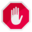## Name

ST_ShiftLongitude — Toggle geometry coordinates between -180..180 and 0..360 ranges.

## Synopsis

`geometry ST_ShiftLongitude(`geometry geomA`)`;

## Description

Reads every point/vertex in every component of every feature in a geometry, and if the longitude coordinate is <0, adds 360 to it. The result would be a 0-360 version of the data to be plotted in a 180 centric mapThis is only useful for data in long lat e.g. 4326 (WGS 84 long lat)Pre-1.3.4 bug prevented this from working for MULTIPOINT. 1.3.4+ works with MULTIPOINT as well.This function supports 3d and will not drop the z-index.

Enhanced: 2.0.0 support for Polyhedral surfaces and TIN was introduced.

NOTE: this function was renamed from "ST_Shift_Longitude" in 2.2.0This function supports Polyhedral surfaces.This function supports Triangles and Triangulated Irregular Network Surfaces (TIN).

## Examples

```--3d points
SELECT ST_AsEWKT(ST_ShiftLongitude(ST_GeomFromEWKT('SRID=4326;POINT(-118.58 38.38 10)'))) As geomA,
ST_AsEWKT(ST_ShiftLongitude(ST_GeomFromEWKT('SRID=4326;POINT(241.42 38.38 10)'))) As geomb
geomA                             geomB
----------                        -----------
SRID=4326;POINT(241.42 38.38 10) SRID=4326;POINT(-118.58 38.38 10)

--regular line string
SELECT ST_AsText(ST_ShiftLongitude(ST_GeomFromText('LINESTRING(-118.58 38.38, -118.20 38.45)')))

st_astext
----------
LINESTRING(241.42 38.38,241.8 38.45)
```